# Civil Engineering - Soil Mechanics and Foundation Engineering

### Exercise :: Soil Mechanics and Foundation Engineering - Section 2

36.

The maximum load carried by a pile, when it continues to sink without further increase of load, is known as

 A. ultimate load carrying capacity B. ultimate bearing capacity C. ultimate bearing resistant D. all the above.

Explanation:

No answer description available for this question. Let us discuss.

37.

The soil which contains finest grain particles, is

 A. coarse sand B. fine sand C. silt D. clay.

Explanation:

No answer description available for this question. Let us discuss.

38.

The general relationship between specific gravity (G), weight of water (γω), degree of saturation (Sr), void ratio (e) and bulk density (γ), is

 A. γ =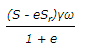B. γ =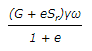C. γ =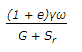D. γ =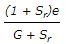Explanation:

No answer description available for this question. Let us discuss.

39.

If C is cohesion, F is factor of safety, γ is unit weight of soil and H is the maximum height of embankment, the stability number, is

 A.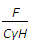B.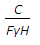C.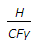D.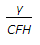E.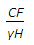Explanation:

No answer description available for this question. Let us discuss.

40.

The angle between the directions of the failure and the major principal plane, is equal to

 A. 90° + effective angle of shearing resistance B. 90° + half of the angle of shearing resistance C. 45° - half of the angle of shearing resistance D. 45° + half of the angle of shearing resistance.